Size of Atoms

The Size of Atoms: Metallic Radii

The size of an isolated atom can't be measured because we can't determine the location of the electrons that surround the nucleus. We can estimate the size of an atom, however, by assuming that the radius of an atom is half the distance between adjacent atoms in a solid. This technique is best suited to elements that are metals, which form solids composed of extended planes of atoms of that element. The results of these measurements are therefore often known as metallic radii.

The figure below shows the relationship between the metallic radii for elements in Groups IA and IIA.There are two general trends in these data.

• The metallic radius becomes larger as we go down a column of the periodic table because the valence electrons are placed in larger orbitals.
• The metallic radius becomes smaller as we go from left to right across a row of the periodic table because the number of protons in the nucleus also increases as we go across a row of the table. The nucleus tends to hold electrons in the same shell of orbitals more tightly and the atoms become smaller.The Size of Atoms: Covalent Radii

The size of an atom can be estimated by measuring the distance between adjacent atoms in a covalent compound. The covalent radius of a chlorine atom, for example, is half the distance between the nuclei of the atoms in a Cl2 molecule.

The covalent radii of the main group elements are given in the figure below. These data confirm the trends observed for metallic radii. Atoms become larger as we go down a column of the periodic table, and they becomes smaller as we go across a row of the table.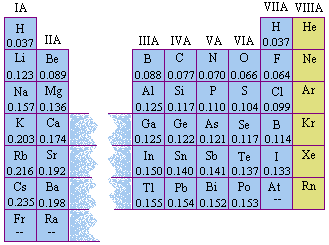The covalent radius for an element is usually a little smaller than the metallic radius. This can be explained by noting that covalent bonds tend to squeeze the atoms together, as shown in the figure below.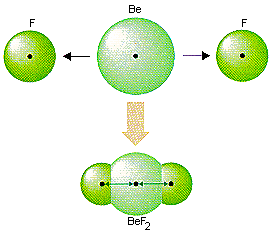The Size of Atoms: Ionic Radii

The relative size of atoms can also be studied by measuring the radii of their ions.

The first ionic radii were obtained by studying the structure of LiI, which contains a relatively small positive ion and a relatively large negative ion. The analysis of the structure of LiI was based on the following assumptions.

• The relatively small Li+ ions pack in the holes between the much larger I- ions, as shown in the figure below.
• The relatively large I- ions touch one another.
• The Li+ ions touch the I- ions.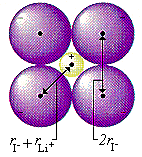If these assumptions are valid, the radius of the I- ion can be estimated by measuring the distance between the nuclei of adjacent iodide ions. The radius of the Li+ ion can then be estimated by subtracting the radius of the I- ion from the distance between the nuclei of adjacent Li+ and I- ions.

Unfortunately only two of the three assumptions that were made for LiI are correct. The Li+ ions in this crystal do not quite touch the I- ions. As a result, this experiment overestimated the size of the Li+ ion. Repeating this analysis with a large number of ionic compounds, however, has made it possible to obtain a set of more accurate ionic radii.The Relative Size of Atoms and Their Ions

The table and figure below compare the covalent radius of neutral F, Cl, Br, and I atoms with the radii of their F-, Cl-, Br-, and I- ions. In each case, the negative ion is much larger than the atom from which it was formed. In fact, the negative ion can be more than twice as large as the neutral atom.

 Element Covalent Radii (nm) Ionic Radii (nm) F 0.064 0.136 Cl 0.099 0.181 Br 0.1142 0.196 I 0.1333 0.216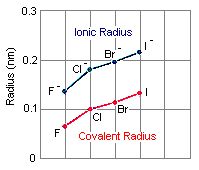The only difference between an atom and its ions is the number of electrons that surround the nucleus.

Example: A neutral chlorine atom contains 17 electrons, while a Cl- ion contains 18 electrons.

 Cl: [Ne] 3s2 3p5 Cl-: [Ne] 3s2 3p6

Because the nucleus can't hold the 18 electrons in the Cl- ion as tightly as the 17 electrons in the neutral atom, the negative ion is significantly larger than the atom from which it forms.

For the same reason, positive ions should be smaller than the atoms from which they are formed. The 11 protons in the nucleus of an Na+ ion, for example, should be able to hold the 10 electrons on this ion more tightly than the 11 electrons on a neutral sodium atom. The table and figure below provide data to test this hypothesis. They compare the covalent radii for neutral atoms of the Group IA elements with the ionic radii for the corresponding positive ions. In each case, the positive ion is much smaller than the atom from which it forms.

 Element Covalent Radii (nm) Ionic Radii (nm) Li 0.123 0.068 Na 0.157 0.095 K 0.2025 0.133 Rb 0.216 0.148 Cs 0.235 0.169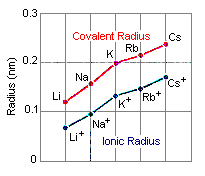Practice Problem 1:Compare the sizes of neutral sodium and chlorine atoms and their Na+ and Cl- ions. Click here to check your answer to Practice Problem 1

The relative size of positive and negative ions has important implications for the structure of ionic compounds. The positive ions are often so small they pack in the holes between planes of adjacent negative ions. In NaCl, for example, the Na+ ions are so small that the Cl- ions almost touch, as shown in the figure below.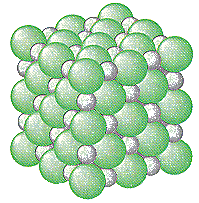Patterns in Ionic Radii

Atoms become larger as we go down a column of the periodic table. We can examine trends in ionic radii across a row of the periodic table by comparing data for atoms and ions that are isoelectronicatoms or ions that have the same number of electrons. The table below summarizes data on the radii of a series of isoelectronic ions and atoms of second- and third-row elements.

Radii for Isoelectronic Second-Row and Third-Row Atoms or Ions

 Atom or Ion Radius (nm) Electron Configuration C4- 0.260 1s2 2s2 2p6 N3- 0.171 1s2 2s2 2p6 O2- 0.140 1s2 2s2 2p6 F- 0.136 1s2 2s2 2p6 Ne 0.112 1s2 2s2 2p6 Na+ 0.095 1s2 2s2 2p6 Mg2+ 0.065 1s2 2s2 2p6 Al3+ 0.050 1s2 2s2 2p6

The data in this table are easy to explain if we note that these atoms or ions all have 10 electrons but the number of protons in the nucleus increases from 6 in the C4- ion to 13 in the Al3+ ion. As the charge on the nucleus becomes larger, the nucleus can hold a constant number of electrons more tightly. As a result, the atoms or ions become significantly smaller.

 Practice Problem 2:Predict which is larger in each of the following pairs of atoms or ions: (a) S2- or O2- (b) Na or Al (c) C4- or F- (d) P3- or P Click here to check your answer to Practice Problem 2